HW 1 Write Up
``library(tidyverse)``

# R4DS

## 3.3.1: 1-4

### 1. What’s gone wrong with this code? Why are the points not blue?

``````ggplot(data = mpg) +
geom_point(mapping = aes(x = displ, y = hwy), color = "blue")``````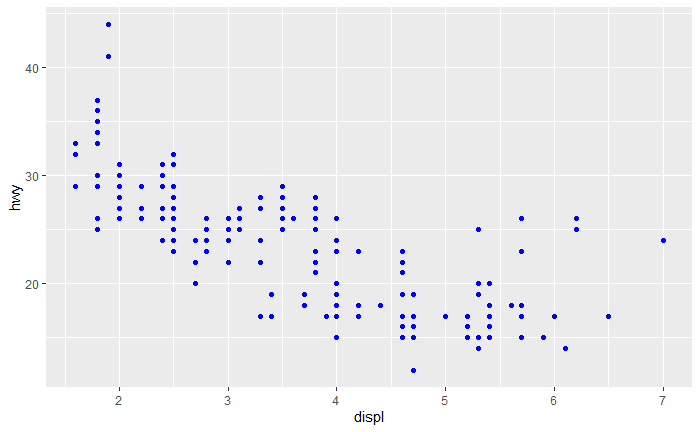color isn’t being used as an aesthetic. It should be outside aes.

### 2. Which variables in mpg are categorical? Which variables are continuous? (Hint: type ?mpg to read the documentation for the dataset). How can you see this information when you run mpg?

``head(mpg)``

Categorical: Manufacturer, Model, Trans, Drv, fl, Class Continuous: hwy, cty, cyl, displ

### 3. Map a continuous variable to color, size, and shape. How do these aesthetics behave differently for categorical vs. continuous variables?

``````ggplot(data = mpg) +
geom_point(mapping = aes(x = displ, y = hwy, size = cty))``````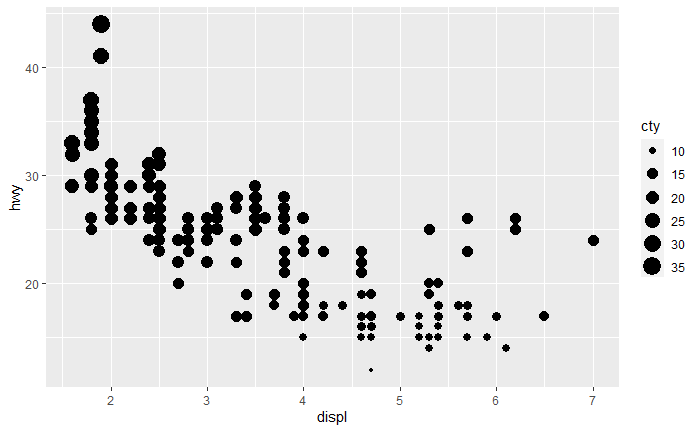Color turns into a gradient Continuous variable won’t map to shape size turns into a gradient

### 4. What happens if you map the same variable to multiple aesthetics?

``````ggplot(data = mpg) +
geom_point(mapping = aes(x = displ, y = hwy, size = hwy, color = hwy))``````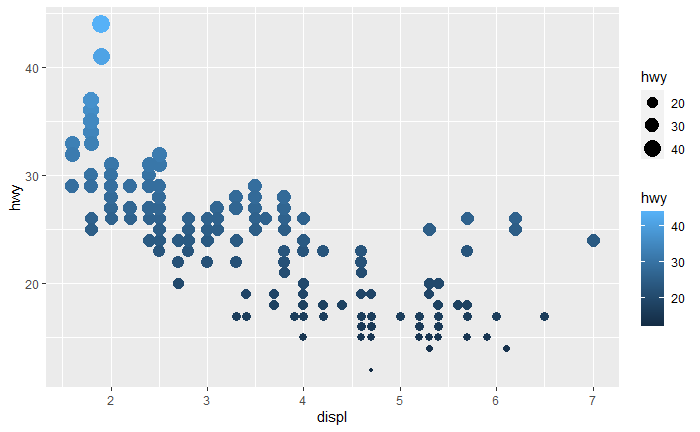I’m not sure exactly what the answer we’re looking for here is. It maps them to multiple aesthetics. This seems potentially useful to emphasize a relationship, but it doesn’t add any new information to the plot.

3.5.1: 1-4, 6

### 1. What happens if you facet on a continuous variable?

``````ggplot(data = mpg) +
geom_point(mapping = aes(x = displ, y = hwy)) +
facet_wrap(~ cty, nrow = 2)``````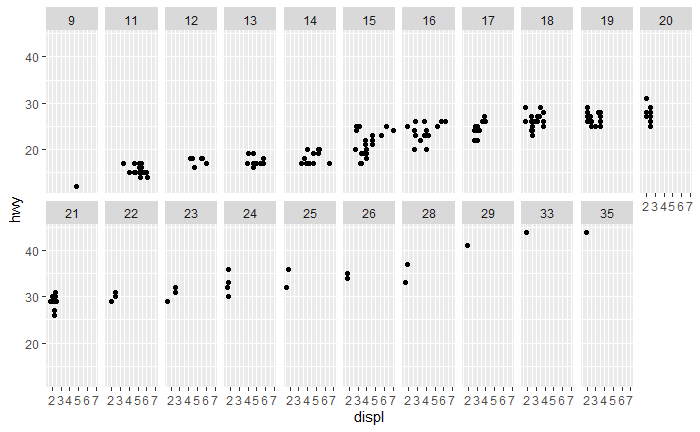Nothing good. It will take each value from your data set and create a plot for it.

### 2. What do the empty cells in plot with facet_grid(drv ~ cyl) mean? How do they relate to this plot?

``````
ggplot(data = mpg) +
geom_point(mapping = aes(x = drv, y = cyl))``````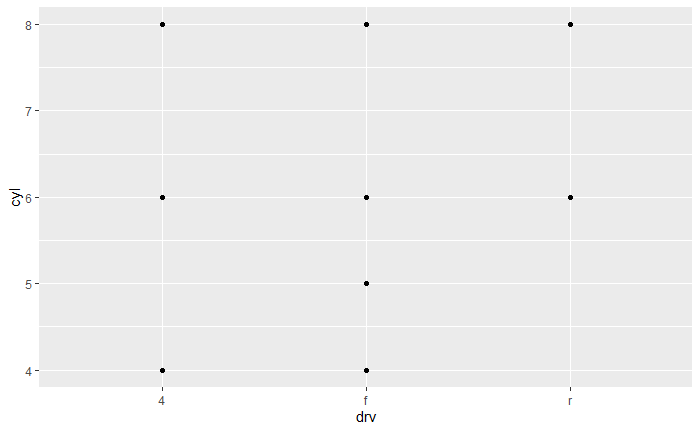``````ggplot(data = mpg) +
geom_point(mapping = aes(x = displ, y = hwy)) +
facet_grid(drv ~ cyl)``````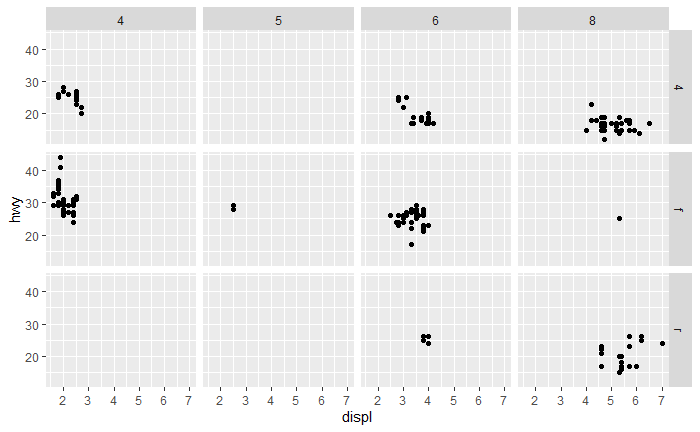Empty cells in facet_grid represent combinations of drv and cyl that don’t have any data in them. You can see which values don’t contain value in the initial plot like the missing points (r,4) and (r,5)

### 3. What plots does the following code make? What does . do?

``````
ggplot(data = mpg) +
geom_point(mapping = aes(x = displ, y = hwy)) +
facet_grid(drv ~ .)``````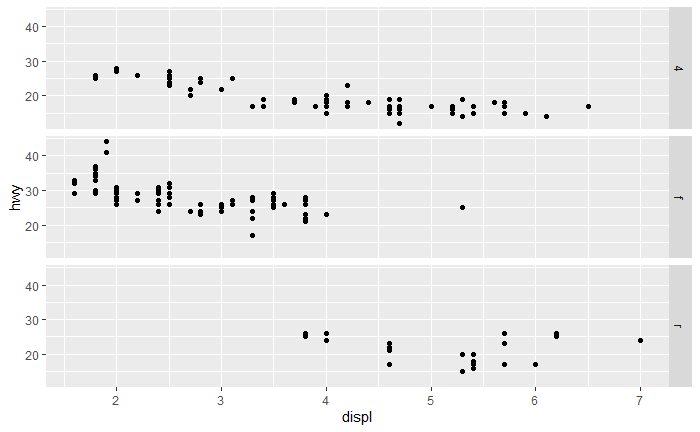``````ggplot(data = mpg) +
geom_point(mapping = aes(x = displ, y = hwy)) +
facet_grid(. ~ cyl)``````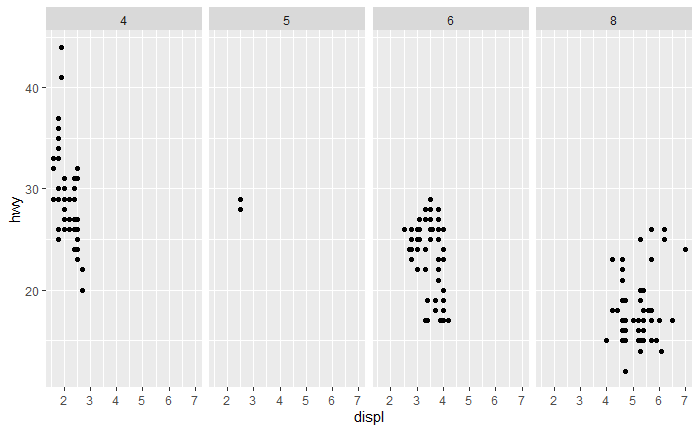The dot creates the facet without an x or y. In the first plot, (drv ~ .) facets by drv on the y axis.

### 4. Take the first faceted plot in this section. What are the advantages to using faceting instead of the colour aesthetic? What are the disadvantages? How might the balance change if you had a larger dataset?

``````ggplot(data = mpg) +
geom_point(mapping = aes(x = displ, y = hwy)) +
facet_wrap(.~class)``````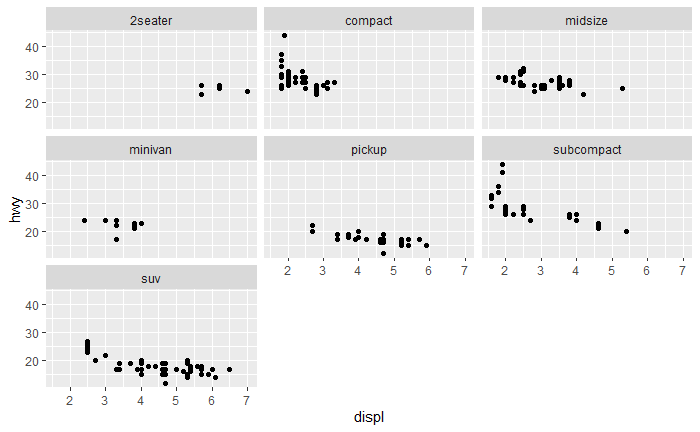``````ggplot(data = mpg) +
geom_point(mapping = aes(x = displ, y = hwy, color = class), position = "jitter")``````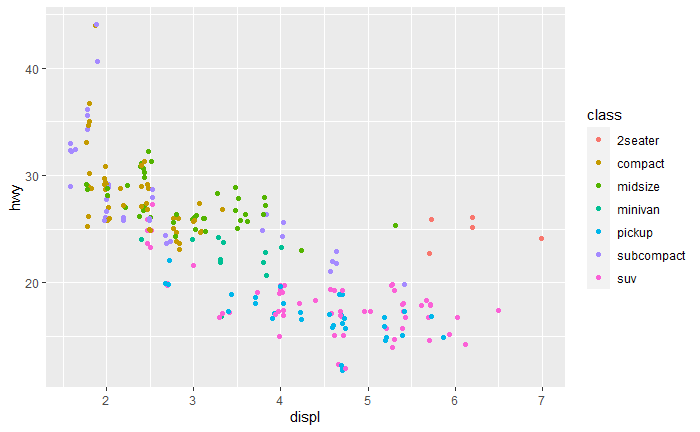In the facet plot, you can clearly see the distribution of each class, while with the color plot it is easy to miss differences in the distributions. Many points are also covered by other points, but jitter can deal with that. The facet plot makes it harder to see overall trends though. As the size of the data set increases, both plots will likely need jitter or some other method for dealing with overlapping points. If the number of levels for class increases, colors will become harder and harder to distinguish.

### 6. When using facet_grid() you should usually put the variable with more unique levels in the columns. Why?

``````ggplot(data = mpg) +
geom_point(mapping = aes(x = displ, y = hwy)) +
facet_grid(cyl~class)``````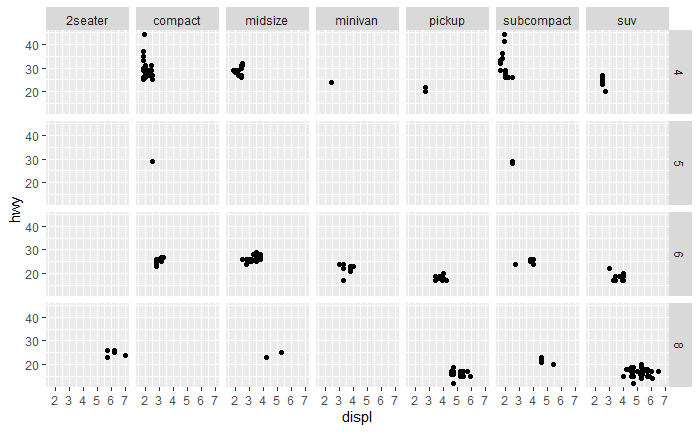``````ggplot(data = mpg) +
geom_point(mapping = aes(x = displ, y = hwy)) +
facet_grid(class~cyl)``````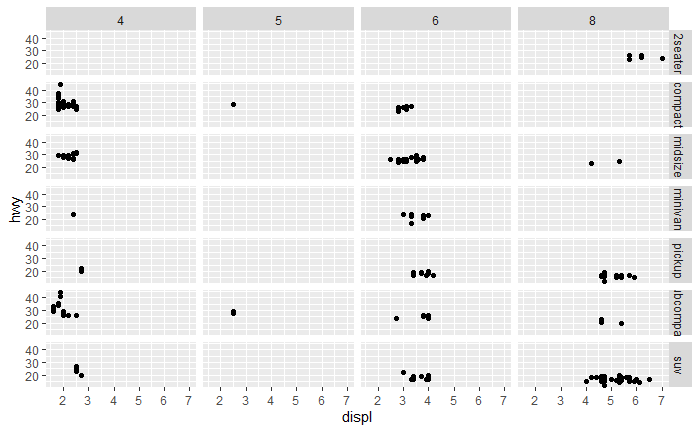Modern computer screens have more horizontal space, so the variable with more levels will have more room in the columns. 3.7.1: 1-5

### 1. What is the default geom associated with stat_summary()? How could you rewrite the previous plot to use that geom function instead of the stat function?

``````ggplot(data = diamonds) +
stat_summary(
mapping = aes(x = cut, y = depth),
fun.min = min,
fun.max = max,
fun = median
)``````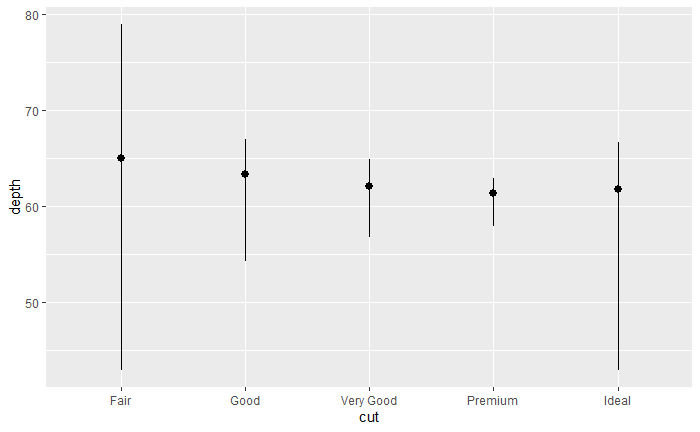Default geom is pointrange

``````# ggplot(data = diamonds) +
#   geom_pointrange(
#     mapping = aes(
#       x = cut, y=median(depth), ymin = min(depth), ymax = max(depth)
#     )
#   )

ggplot(data = diamonds) +
geom_pointrange(
mapping = aes(x = cut, y = depth),
stat = "summary",
fun.min = min,
fun.max = max,
fun = median
)``````### 2. What does geom_col() do? How is it different to geom_bar()?

From the description,

There are two types of bar charts: geom_bar() and geom_col(). geom_bar() makes the height of the bar proportional to the number of cases in each group (or if the weight aesthetic is supplied, the sum of the weights). If you want the heights of the bars to represent values in the data, use geom_col() instead

``````ggplot(data = diamonds) +
geom_bar(mapping = aes(x = cut))``````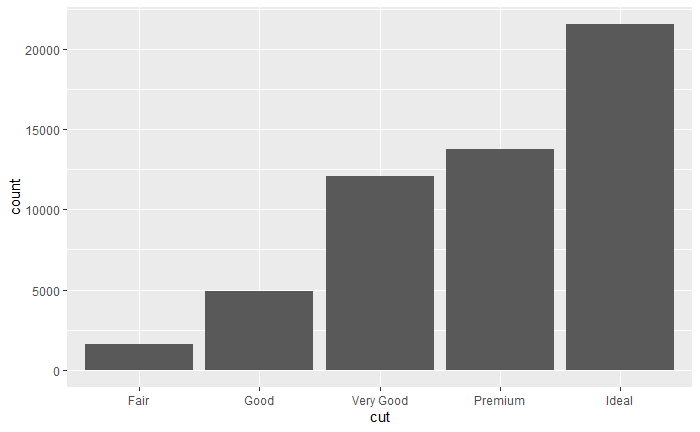``````ggplot(data = diamonds) +
geom_col(mapping = aes(x = cut, y = price))``````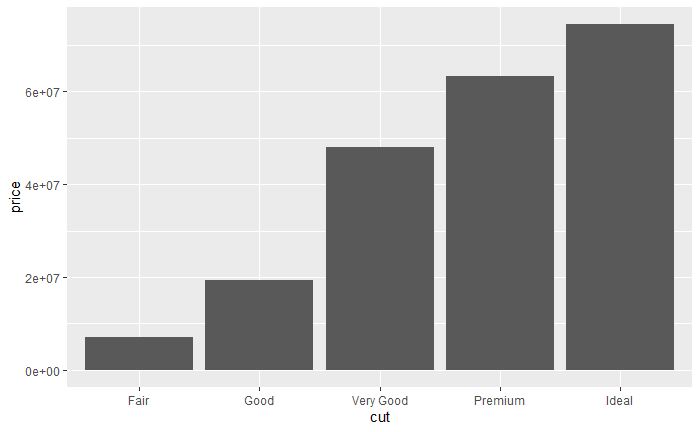These graphs may look similar, but realize that for the 2nd price the height is the sum of the price for each data point split by cut.

### 3. Most geoms and stats come in pairs that are almost always used in concert. Read through the documentation and make a list of all the pairs. What do they have in common?

There are a bunch so I won’t list them, but they can be found at https://ggplot2.tidyverse.org/reference/ … except that this is a submitted problem so I guess I will

Geom Stat Pairs
Geom Stat
geom_bar() stat_count()
geom_bin2d() stat_bin_2d()
geom_boxplot() stat_boxplot()
geom_contour() stat_contour()
geom_count() stat_sum()
geom_density() stat_density()
geom_histogram() stat_bin()
geom_qq_line() stat_qq_line()
geom_quantile() stat_quantile()
geom_smooth() stat_smooth()
geom_function() stat_function()

They tend to have the same name like geom_boxplot() stat_boxplot()

### 4. What variables does stat_smooth() compute? What parameters control its behaviour?

Stat_smooth() computes y, the predicted value, ymin, ymax, and standard error. It is controled by the following:

Method - Function used for smoothing Formula - Formula to use in smoothing function Se - Display the CI around smooth

### 5. In our proportion bar chart, we need to set group = 1. Why? In other words what is the problem with these two graphs?

``````ggplot(data = diamonds) +
geom_bar(mapping = aes(x = cut, y = after_stat(prop)))``````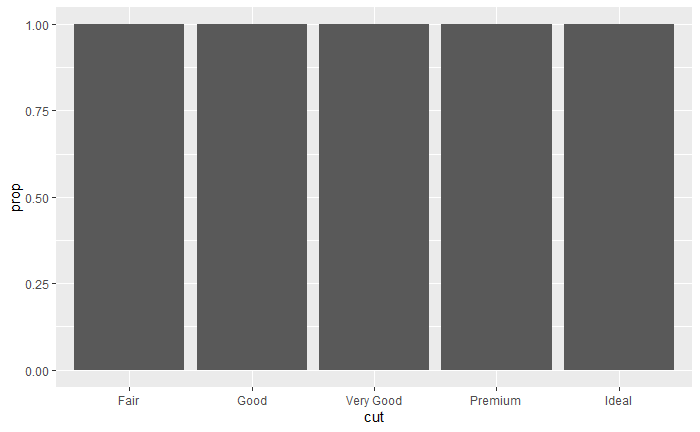``````ggplot(data = diamonds) +
geom_bar(mapping = aes(x = cut, fill = color, y = after_stat(prop)))``````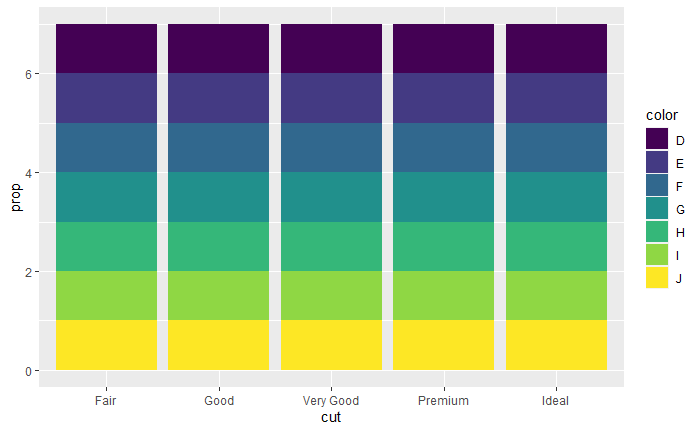Both plots aren’t actually proportional. Each level is made proportional accross the board, rather than by each level. First one needs group = 1 and the 2nd needs

``````ggplot(data = diamonds) +
geom_bar(mapping = aes(x = cut, y = after_stat(prop), group = 1))``````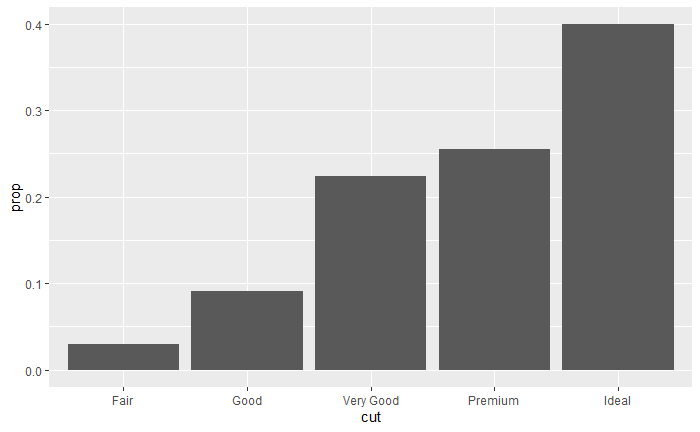``````ggplot(data = diamonds) +
geom_bar(mapping = aes(x = cut, fill = color, y = ..count../sum(..count..), group = color))``````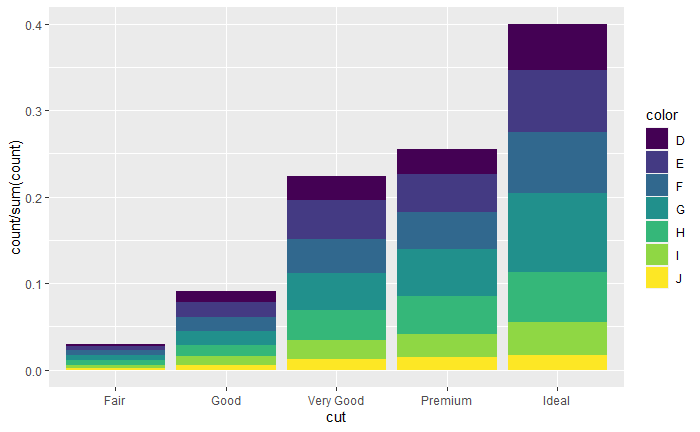# Introduction to Statistical Learning

## 2.4: 1-3, 7, 8, 10

### 1. For each of parts (a) through (d), indicate whether we would generally expect the performance of a flexible statistical learning method to be better or worse than an inflexible method. Justify your answer.

#### (a) The sample size n is extremely large, and the number of predictors p is small.

Flexible should perform well since the data set is very large, but so should an inflexible method since the number of predictors is small.

#### (b) The number of predictors p is extremely large, and the number of observations n is small.

Both will perform poorly, but flexible method is bound to over fit and be really bad since n is small

#### (c) The relationship between the predictors and response is highly non-linear.

Flexible should perform better? I’m not sure about this one… there are inflexible models that are highly non-linear

#### (d) The variance of the error terms, i.e. σ2 = Var(ϵ), is extremely high

Inflexible, since var(e) is very high, flexible methods will chase those outliers that will be far from the true relationship.

### 2. Explain whether each scenario is a classification or regression problem, and indicate whether we are most interested in inference or prediction. Finally, provide n and p.

#### (a) We collect a set of data on the top 500 firms in the US. For each firm we record profit, number of employees, industry and the CEO salary. We are interested in understanding which factors affect CEO salary

Regression, inference n = 500 firms p = employees, industry, profit

#### (b) We are considering launching a new product and wish to know whether it will be a success or a failure. We collect data on 20 similar products that were previously launched. For each product we have recorded whether it was a success or failure, price charged for the product, marketing budget, competition price. and ten other variables

Classification, prediction n = 20 similar products p = Price charged, budget, competition price, 10 other variables

#### (c) We are interested in predicting the % change in the USD/Euro exchange rate in relation to the weekly changes in the world stock markets. Hence we collect weekly data for all of 2012. For each week we record the % change in the USD/Euro, the % change in the US market, the % change in the British market, and the % change in the German market

Regression, prediction n = number of weeks in 2012, 52 p = change US, change British, change German

### 3. We now revisit the bias-variance decomposition.

#### (b) Explain why each of the five curves has the shape displayed in part (a).

Variance will always increase as we increase flexibility since more flexible models are more “wiggly” by definition Bias will always decrease as we increase flexibility since more flexible models approach the true values at points in the data This same reasoning applies to training error The test error will decrease until over fitting at which point it will increase Bayes error is the irreducible error and is the same no matter the flexibility

### 7. The table below provides a training data set containing six observations, three predictors, and one qualitative response variable. Suppose we wish to use this data set to make a prediction for Y when X1 = X2 = X3 = 0 using K-nearest neighbors.

``````x1 = c(0,2,0,0,-1,1)
x2 = c(3,0,1,1,0,1)
x3 = c(0,0,3,2,1,1)
y = c(0,0,0,1,1,0)

table7= tibble(x1,x2,x3,y)
typeof(table7\$x1)``````
`` "double"``

#### (a) Compute the Euclidean distance between each observation and the test point, X1 = X2 = X3 = 0.

``````x0 = c(0,0,0)
dist.c <- function(x1,x2,x3){
sum((c(x1,x2,x3)-x0)**2)
}
#dist.c(table7[,1],table7[,2],table7[,3])
table7%>%
mutate(dist.to.x=pmap_dbl(list(table7\$x1,table7\$x2,table7\$x3),dist.c))%>%#wow that took a lon time to figure out. I feels silly.
arrange(dist.to.x)``````
``NA``

#### (b) What is our prediction with K = 1? Why?

Green, the closest 1 is green.

#### (c) What is our prediction with K = 3? Why?

Red. Closest three have green green red so there are more greens then red.

#### (d) If the Bayes decision boundary in this problem is highly nonlinear, then would we expect the best value for K to be large or small? Why?

Small. As we saw in Thursday lecture, a large K value will create a line averaging the sets.

### 8. This exercise relates to the College data set, which can be found in the file College.csv. It contains a number of variables for 777 different universities and colleges in the US.

#### (a) Use the read.csv() function to read the data into R. Call the loaded data college. Make sure that you have the directory set to the correct location for the data

``````college = read.csv("College.csv")

#### (b) Look at the data using the fix() function. You should notice that the first column is just the name of each university. We don’t really want R to treat this as data. However, it may be handy to have these names for later.

``````rownames(college) = college[,1]
fix(college)
college =college [,-1]
fix(college)``````

#### (c)

``summary(college)``
``````   Private               Apps           Accept          Enroll       Top10perc       Top25perc      F.Undergrad     P.Undergrad         Outstate
Length:777         Min.   :   81   Min.   :   72   Min.   :  35   Min.   : 1.00   Min.   :  9.0   Min.   :  139   Min.   :    1.0   Min.   : 2340
Class :character   1st Qu.:  776   1st Qu.:  604   1st Qu.: 242   1st Qu.:15.00   1st Qu.: 41.0   1st Qu.:  992   1st Qu.:   95.0   1st Qu.: 7320
Mode  :character   Median : 1558   Median : 1110   Median : 434   Median :23.00   Median : 54.0   Median : 1707   Median :  353.0   Median : 9990
Mean   : 3002   Mean   : 2019   Mean   : 780   Mean   :27.56   Mean   : 55.8   Mean   : 3700   Mean   :  855.3   Mean   :10441
3rd Qu.: 3624   3rd Qu.: 2424   3rd Qu.: 902   3rd Qu.:35.00   3rd Qu.: 69.0   3rd Qu.: 4005   3rd Qu.:  967.0   3rd Qu.:12925
Max.   :48094   Max.   :26330   Max.   :6392   Max.   :96.00   Max.   :100.0   Max.   :31643   Max.   :21836.0   Max.   :21700
Room.Board       Books           Personal         PhD            Terminal       S.F.Ratio      perc.alumni        Expend        Grad.Rate
Min.   :1780   Min.   :  96.0   Min.   : 250   Min.   :  8.00   Min.   : 24.0   Min.   : 2.50   Min.   : 0.00   Min.   : 3186   Min.   : 10.00
1st Qu.:3597   1st Qu.: 470.0   1st Qu.: 850   1st Qu.: 62.00   1st Qu.: 71.0   1st Qu.:11.50   1st Qu.:13.00   1st Qu.: 6751   1st Qu.: 53.00
Median :4200   Median : 500.0   Median :1200   Median : 75.00   Median : 82.0   Median :13.60   Median :21.00   Median : 8377   Median : 65.00
Mean   :4358   Mean   : 549.4   Mean   :1341   Mean   : 72.66   Mean   : 79.7   Mean   :14.09   Mean   :22.74   Mean   : 9660   Mean   : 65.46
3rd Qu.:5050   3rd Qu.: 600.0   3rd Qu.:1700   3rd Qu.: 85.00   3rd Qu.: 92.0   3rd Qu.:16.50   3rd Qu.:31.00   3rd Qu.:10830   3rd Qu.: 78.00
Max.   :8124   Max.   :2340.0   Max.   :6800   Max.   :103.00   Max.   :100.0   Max.   :39.80   Max.   :64.00   Max.   :56233   Max.   :118.00  ``````
``pairs(college[,2:10]) #This is a terrible picture. Can gg plot do this?``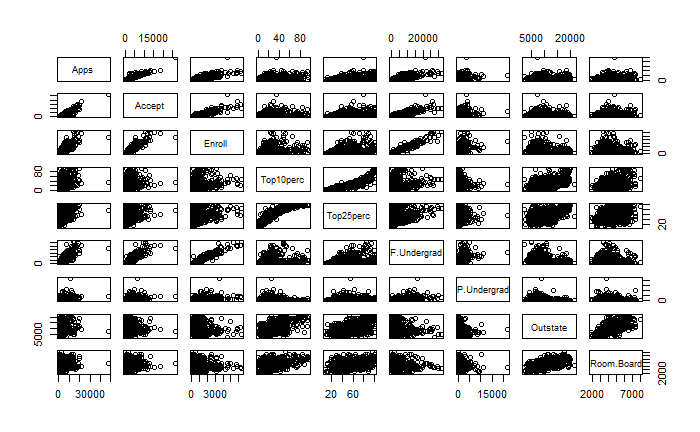``````ggplot(data = college) +
geom_boxplot(mapping = aes(x = Private, y = Outstate))``````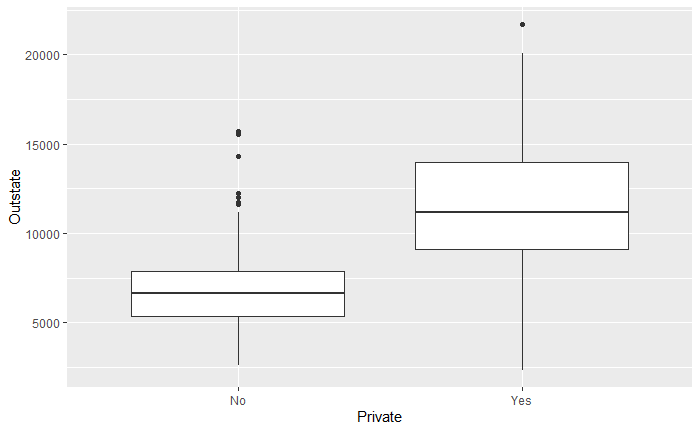``````Elite=rep("No",nrow(college ))
Elite[college\$Top10perc >50]=" Yes"
Elite=as.factor(Elite)
college=data.frame( college , Elite)
summary(college)``````
``````   Private               Apps           Accept          Enroll       Top10perc       Top25perc      F.Undergrad     P.Undergrad         Outstate
Length:777         Min.   :   81   Min.   :   72   Min.   :  35   Min.   : 1.00   Min.   :  9.0   Min.   :  139   Min.   :    1.0   Min.   : 2340
Class :character   1st Qu.:  776   1st Qu.:  604   1st Qu.: 242   1st Qu.:15.00   1st Qu.: 41.0   1st Qu.:  992   1st Qu.:   95.0   1st Qu.: 7320
Mode  :character   Median : 1558   Median : 1110   Median : 434   Median :23.00   Median : 54.0   Median : 1707   Median :  353.0   Median : 9990
Mean   : 3002   Mean   : 2019   Mean   : 780   Mean   :27.56   Mean   : 55.8   Mean   : 3700   Mean   :  855.3   Mean   :10441
3rd Qu.: 3624   3rd Qu.: 2424   3rd Qu.: 902   3rd Qu.:35.00   3rd Qu.: 69.0   3rd Qu.: 4005   3rd Qu.:  967.0   3rd Qu.:12925
Max.   :48094   Max.   :26330   Max.   :6392   Max.   :96.00   Max.   :100.0   Max.   :31643   Max.   :21836.0   Max.   :21700
Room.Board       Books           Personal         PhD            Terminal       S.F.Ratio      perc.alumni        Expend        Grad.Rate
Min.   :1780   Min.   :  96.0   Min.   : 250   Min.   :  8.00   Min.   : 24.0   Min.   : 2.50   Min.   : 0.00   Min.   : 3186   Min.   : 10.00
1st Qu.:3597   1st Qu.: 470.0   1st Qu.: 850   1st Qu.: 62.00   1st Qu.: 71.0   1st Qu.:11.50   1st Qu.:13.00   1st Qu.: 6751   1st Qu.: 53.00
Median :4200   Median : 500.0   Median :1200   Median : 75.00   Median : 82.0   Median :13.60   Median :21.00   Median : 8377   Median : 65.00
Mean   :4358   Mean   : 549.4   Mean   :1341   Mean   : 72.66   Mean   : 79.7   Mean   :14.09   Mean   :22.74   Mean   : 9660   Mean   : 65.46
3rd Qu.:5050   3rd Qu.: 600.0   3rd Qu.:1700   3rd Qu.: 85.00   3rd Qu.: 92.0   3rd Qu.:16.50   3rd Qu.:31.00   3rd Qu.:10830   3rd Qu.: 78.00
Max.   :8124   Max.   :2340.0   Max.   :6800   Max.   :103.00   Max.   :100.0   Max.   :39.80   Max.   :64.00   Max.   :56233   Max.   :118.00
Elite     Elite.1
Yes: 78    Yes: 78
No  :699   No  :699

``````
``````ggplot(data = college) +
geom_boxplot(mapping = aes(x = Elite, y = Outstate))``````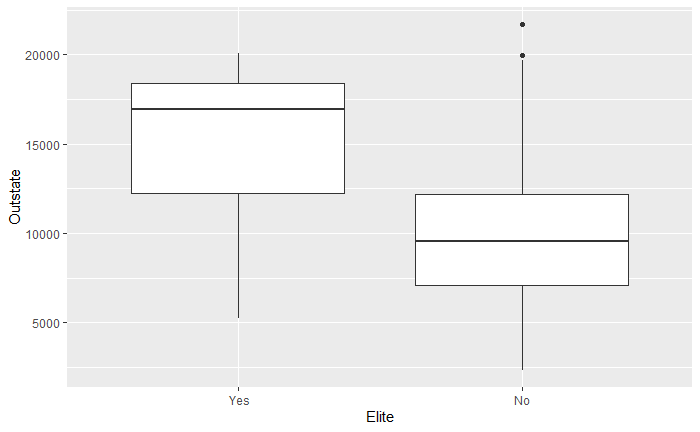``````ggplot(data = college) +
geom_histogram(mapping = aes(x = Outstate),bins = 50)``````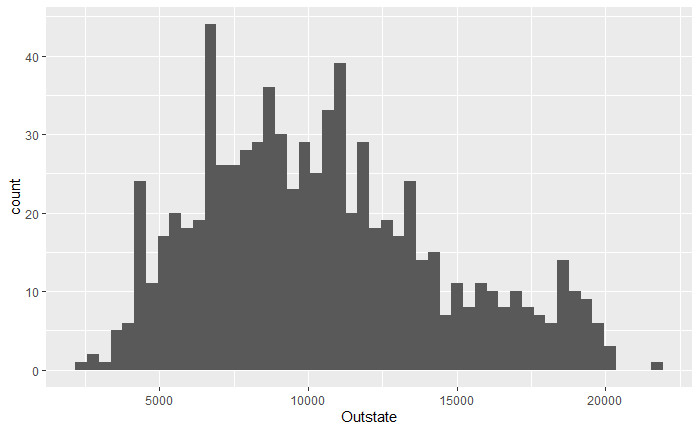``````ggplot(data = college) +
geom_histogram(mapping = aes(x = Outstate),bins = 10)``````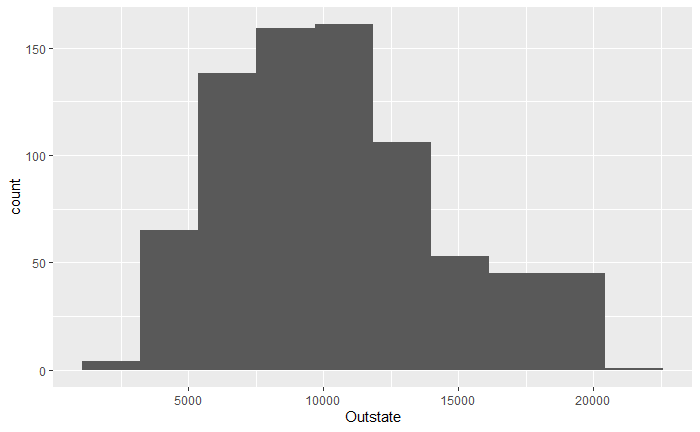``````ggplot(data = college) +
geom_histogram(mapping = aes(x = Accept),bins = 100)``````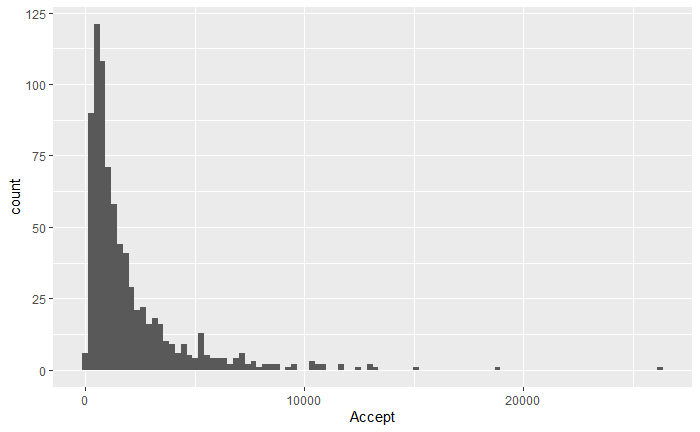``````ggplot(data = college) +
geom_boxplot(mapping = aes(x = Private, y = Grad.Rate))``````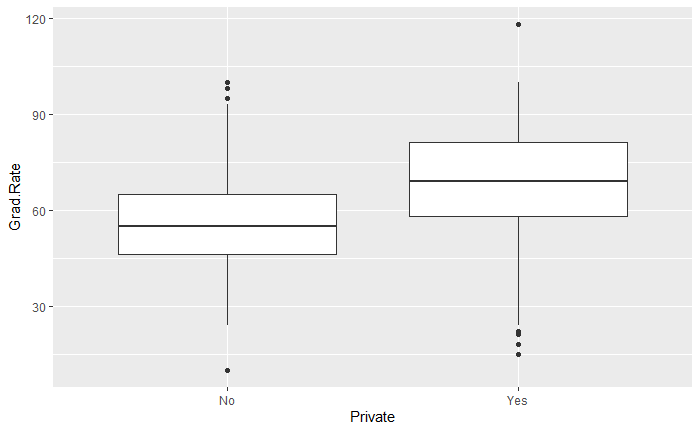Private schools have a much higher graduation rate. Could it be that there is some sort of pressure to pass in private schools!?

``````ggplot(data = college) +
geom_boxplot(mapping = aes(x = Private, y = Expend))``````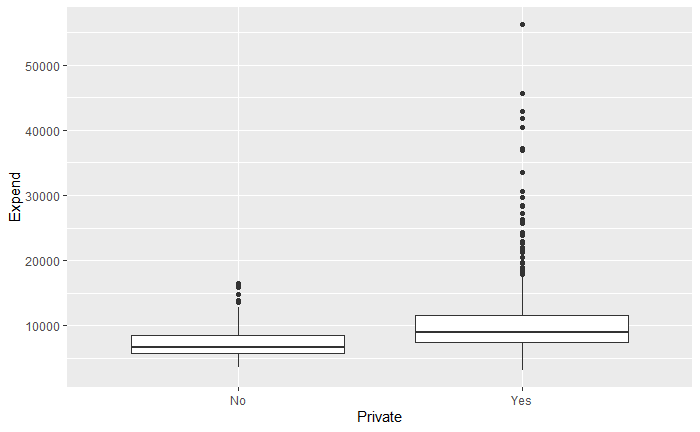Not really a surprise, but private schools spend more per student and the variance of what they spend is much higher.

## 3.7: 3, 10

### 3. Suppose we have a data set with five predictors, X1 = GPA, X2 = IQ, X3 = Gender (1 for Female and 0 for Male), X4 = Interaction between GPA and IQ, and X5 = Interaction between GPA and Gender. The response is starting salary after graduation (in thousands of dollars). Suppose we use least squares to fit the model, and get βˆ0 = 50, βˆ1 = 20, βˆ2 = 0.07, βˆ3 = 35, βˆ4 = 0.01, βˆ5 = −10.

#### (a) Which answer is correct, and why?fgv

i. For a fixed value of IQ and GPA, males earn more on average than females.

ii. For a fixed value of IQ and GPA, females earn more on average than males.

iii. For a fixed value of IQ and GPA, males earn more on average than females provided that the GPA is high enough.

True! if we consider the function y = 50 + 20 * gpa + .07 * iq + 35 * gender + .01 * (gpa * iq) -10 * (gpa * gender) so if we have an iq of 100, the interaction term will subtract 100 while being a women only adds 35.

iv. For a fixed value of IQ and GPA, females earn more on average than males provided that the GPA is high enough.

#### (b) Predict the salary of a female with IQ of 110 and a GPA of 4.0.

``50+20*4+.07*110+35*1+.01*(4 * 110) -10*(4*1)``
`` 137.1``

#### (c) True or false: Since the coefficient for the GPA/IQ interaction term is very small, there is very little evidence of an interaction effect. Justify your answer.

False, there could be an interaction effect if the pval is small, but it just doesn’t have a very large coefficient.

### 10. This question should be answered using the Carseats data set.

``````library(ISLR)
data("Carseats")
car = Carseats
car``````

#### (a) Fit a multiple regression model to predict Sales using Price, Urban, and US.

``summary(model1 <- lm(Sales~Price + Urban + US, data = car))``
``````
Call:
lm(formula = Sales ~ Price + Urban + US, data = car)

Residuals:
Min      1Q  Median      3Q     Max
-6.9206 -1.6220 -0.0564  1.5786  7.0581

Coefficients:
Estimate Std. Error t value Pr(>|t|)
(Intercept) 13.043469   0.651012  20.036  < 2e-16 ***
Price       -0.054459   0.005242 -10.389  < 2e-16 ***
UrbanYes    -0.021916   0.271650  -0.081    0.936
USYes        1.200573   0.259042   4.635 4.86e-06 ***
---
Signif. codes:  0 ‘***’ 0.001 ‘**’ 0.01 ‘*’ 0.05 ‘.’ 0.1 ‘ ’ 1

Residual standard error: 2.472 on 396 degrees of freedom
Multiple R-squared:  0.2393,    Adjusted R-squared:  0.2335
F-statistic: 41.52 on 3 and 396 DF,  p-value: < 2.2e-16``````

#### (c) Write out the model in equation form, being careful to handle the qualitative variables properly.

Sales = 13.043469 - .054459 * Price - 0.021916 (if urban) + 1.200573 (if US)

Price, US

#### (e) On the basis of your response to the previous question, fit a smaller model that only uses the predictors for which there is evidence of association with the outcome.

``summary(model2 <- lm(Sales~Price + US, data = car))``
``````
Call:
lm(formula = Sales ~ Price + US, data = car)

Residuals:
Min      1Q  Median      3Q     Max
-6.9269 -1.6286 -0.0574  1.5766  7.0515

Coefficients:
Estimate Std. Error t value Pr(>|t|)
(Intercept) 13.03079    0.63098  20.652  < 2e-16 ***
Price       -0.05448    0.00523 -10.416  < 2e-16 ***
USYes        1.19964    0.25846   4.641 4.71e-06 ***
---
Signif. codes:  0 ‘***’ 0.001 ‘**’ 0.01 ‘*’ 0.05 ‘.’ 0.1 ‘ ’ 1

Residual standard error: 2.469 on 397 degrees of freedom
Multiple R-squared:  0.2393,    Adjusted R-squared:  0.2354
F-statistic: 62.43 on 2 and 397 DF,  p-value: < 2.2e-16``````

#### (f) How well do the models in (a) and (e) fit the data?

Very poorly. R squ is terrible for both models.

#### (g) Using the model from (e), obtain 95 % confidence intervals for the coefficient(s).

``confint(model2)``
``````                  2.5 %      97.5 %
(Intercept) 11.79032020 14.27126531
Price       -0.06475984 -0.04419543
USYes        0.69151957  1.70776632``````

#### (h) Is there evidence of outliers or high leverage observations in the model from (e)?

``````par(mfrow=c(2,2))
plot(model2)``````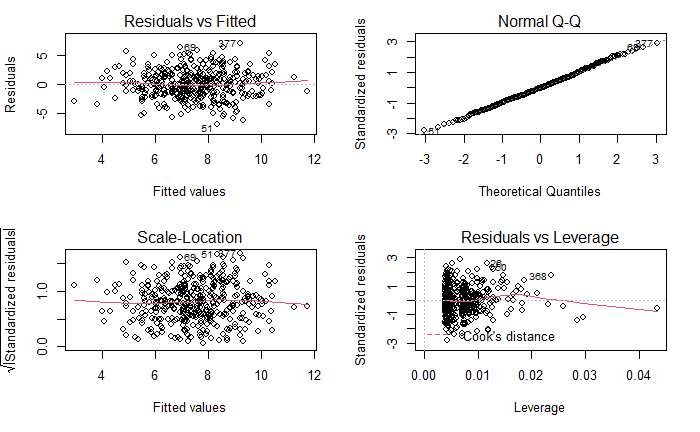No.

## 4.7: 1, 3, 4, 9, 10a-d

### 1. Using a little bit of algebra, prove that (4.2) is equivalent to (4.3). In other words, the logistic function representation and logit representation for the logistic regression model are equivalent.

This is straight forward and I’d have to insert a picture so…

### 3. This problem relates to the QDA model, in which the observations within each class are drawn from a normal distribution with a classspecific mean vector and a class specific covariance matrix. We consider the simple case where p = 1; i.e. there is only one feature. Suppose that we have K classes, and that if an observation belongs to the kth class then X comes from a one-dimensional normal distribution, X ∼ N(µk, σ2 k). Recall that the density function for the one-dimensional normal distribution is given in (4.11). Prove that in this case, the Bayes’ classifier is not linear. Argue that it is in fact quadratic. Hint: For this problem, you should follow the arguments laid out in Section 4.4.2, but without making the assumption that σ^21 = … = σ^2K.

We end up with a x^2 from the expansion that is multiplied by sigma_k which must stay in our equation since it is in terms of k.

### 4. When the number of features p is large, there tends to be a deterioration in the performance of KNN and other local approaches that perform prediction using only observations that are near the test observation for which a prediction must be made. This phenomenon is known as the curse of dimensionality, and it ties into the fact that curse of dinon-parametric approaches often perform poorly when p is large. We will now investigate this curse.

10%

10% * 10% = 1%

#### (c) Now suppose that we have a set of observations on p = 100 features. Again the observations are uniformly distributed on each feature, and again each feature ranges in value from 0 to 1. We wish to predict a test observation’s response using observations within the 10 % of each feature’s range that is closest to that test observation. What fraction of the available observations will we use to make the prediction?

10%^100

``.1**100``
`` 1e-100``

#### (d) Using your answers to parts (a)–(c), argue that a drawback of KNN when p is large is that there are very few training observations “near” any given test observation.

As p increases, the number of of “near” observations decreases exponentially.

#### (e) Now suppose that we wish to make a prediction for a test observation by creating a p-dimensional hypercube centered around the test observation that contains, on average, 10 % of the training observations. For p = 1, 2, and 100, what is the length of each side of the hypercube? Comment on your answer.

Note: A hypercube is a generalization of a cube to an arbitrary number of dimensions. When p = 1, a hypercube is simply a line segment, when p = 2 it is a square, and when p = 100 it is a 100-dimensional cube.

p = 1: .1 p = 2:

``.1**(1/2)``
`` 0.3162278``

p=100:

``.1**(1/100)``
`` 0.9772372``

As p increases, we need more and more data. When p = 100, we need to use almost our entire data set.

### 9. This problem has to do with odds

#### (a) On average, what fraction of people with an odds of 0.37 of defaulting on their credit card payment will in fact default?

``.37/(.37+1)``
`` 0.270073``

#### (b) Suppose that an individual has a 16 % chance of defaulting on her credit card payment. What are the odds that she will default?

.16 = odds/(1+odds) .16 + .16odds = odds .16 = .84odds odds = .16/.84

``.16/.84``
`` 0.1904762``

Simply

``.16/(1-.16)``
`` 0.1904762``